Courses

# Test: Graphs Theory- 1

## 20 Questions MCQ Test GATE Computer Science Engineering(CSE) 2022 Mock Test Series | Test: Graphs Theory- 1

Description
This mock test of Test: Graphs Theory- 1 for Computer Science Engineering (CSE) helps you for every Computer Science Engineering (CSE) entrance exam. This contains 20 Multiple Choice Questions for Computer Science Engineering (CSE) Test: Graphs Theory- 1 (mcq) to study with solutions a complete question bank. The solved questions answers in this Test: Graphs Theory- 1 quiz give you a good mix of easy questions and tough questions. Computer Science Engineering (CSE) students definitely take this Test: Graphs Theory- 1 exercise for a better result in the exam. You can find other Test: Graphs Theory- 1 extra questions, long questions & short questions for Computer Science Engineering (CSE) on EduRev as well by searching above.
QUESTION: 1

Solution:
QUESTION: 2

Solution:
QUESTION: 3

### The line graph L(G) of a simple graph G is defined as follows: · There is exactly one vertex v(e) in L(G) for each edge e in G. · For any two edges e and e' in G, L(G) has an edge between v(e) and v(e'), if and only if e and e'are incident with the same vertex in G. Which of the following statements is/are TRUE? (P) The line graph of a cycle is a cycle. (Q) The line graph of a clique is a clique. (R) The line graph of a planar graph is planar. (S) The line graph of a tree is a tree.

Solution:
QUESTION: 4

Let G be a simple undirected planar graph on 10 vertices with 15 edges. If G is a connected graph, then the number of bounded faces in any embedding of G on the plane is equal to

Solution:

If the graph is planar, then it must follow below Euler's Formula for planar graphs

v - e + f = 2
v is number of vertices
e is number of edges
f is number of faces including bounded and unbounded

10 - 15 + f = 2
f = 7
There is always one unbounded face, so the number of bounded faces =  6

QUESTION: 5

Which of the following graphs is isomorphic to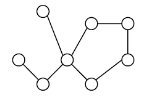Solution:

See Graph isomorphism

QUESTION: 6

Let G be a complete undirected graph on 6 vertices. If vertices of G are labeled, then the number of distinct cycles of length 4 in G is equal to

Solution:

There can be total 6C4 ways to pick 4 vertices from 6. The value of 6C4 is 15. Note that the given graph is complete so any 4 vertices can form a cycle. There can be 6 different cycle with 4 vertices. For example, consider 4 vertices as a, b, c and d. The three distinct cycles are cycles should be like this (a, b, c, d,a) (a, b, d, c,a) (a, c, b, d,a) (a, c, d, b,a) (a, d, b, c,a) (a, d, c, b,a) and (a, b, c, d,a) and (a, d, c, b,a) (a, b, d, c,a) and (a, c, d, b,a) (a, c, b, d,a) and (a, d, b, c,a) are same cycles. So total number of distinct cycles is (15*3) = 45. **NOTE**: In original GATE question paper 45 was not an option. In place of 45, there was 90.

QUESTION: 7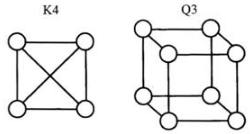Solution:

A Graph is said to be planar if it can be drawn in a plane without any edges crossing each other. Following are planar embedding of the given two graph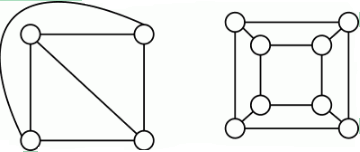QUESTION: 8

Let G = (V,E) be a graph. Define ξ(G) = Σd id x d, where id is the number of vertices of degree d in G. If S and T are two different trees with ξ(S) = ξ(T),then

Solution:

The expression ξ(G) is basically sum of all degrees in a tree.   For example, in the following tree, the sum is 3 + 1 + 1 + 1.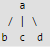Now the questions is, if sum of degrees in trees are same, then what is the relationship between number of vertices present in both trees? The answer is, ξ(G) and ξ(T) is same for two trees, then the trees have same number of vertices. It can be proved by induction. Let it be true for n vertices. If we add a vertex, then the new vertex (if it is not the first node) increases degree by 2, it doesn't matter where we add it. For example, try to add a new vertex say 'e' at different places in above example tee.

QUESTION: 9

The degree sequence of a simple graph is the sequence of the degrees of the nodes in the graph in decreasing order. Which of the following sequences can not be the degree sequence of any graph?

I. 7, 6, 5, 4, 4, 3, 2, 1
II. 6, 6, 6, 6, 3, 3, 2, 2
III. 7, 6, 6, 4, 4, 3, 2, 2
IV. 8, 7, 7, 6, 4, 2, 1, 1

Solution:

A generic algorithm or method to solve this question is 1: procedure isV alidDegreeSequence(L) 2: for n in list L do 3: if L doesn’t have n elements next to the current one then return false 4: decrement next n elements of the list by 1 5: arrange it back as a degree sequence, i.e. in descending order 6: if any element of the list becomes negative then return false 7: return true Rationale behind this method comes from the properties of simple graph. Enumerating the f alse returns, 1) if L doesn’t have enough elements after the current one or 2) if any element of the list becomes negative, then it means that there aren’t enough nodes to accommodate edges in a simple graph fashion, which will lead to violation of either of the two conditions of the simple graph (no self-loops and no multiple-edges between two nodes), if not others.

: → According to this theorem, Let D be sequence the d1,d2,d2. . . dn with d1 ≥ d2 ≥ d2 ≥ . . . dn for n≥ 2 and di ≥ 0. → Then D0 be the sequence obtained by: → Discarding d1, and → Subtracting 1 from each of the next d1 entries of D. → That is Degree sequence D0 would be : d2-1, d2-1, d3-1 . . . , dd1+1 -1 . . . , dn → Then, D is graphical if and only if D0 is graphical. Now, we apply this theorem to given sequences: option I) 7,6,5,4,4,3,2,1 → 5,4,3,3,2,1,0 → 3,2,2,1,0,0 → 1,1,0,0,0 → 0,0,0,0 so its graphical. Option II) 6,6,6,6,3,3,2,2 → 5,5,5,2,2,1,2 ( arrange in ascending order) → 5,5,5,2,2,2,1 → 4,4,1,1,1,0 → 3,0,0,0,0 → 2,-1,-1,-1,0 but d (degree of a vertex) is non negative so its not a graphical. Option III) 7,6,6,4,4,3,2,2 → 5,5,3,3,2,1,1 → 4,2,2,1,1,0 → 1,1,0,0,0 → 0,0,0,0 so its graphical. Option IV) 8,7,7,6,4,2,1,1 , here degree of a vertex is 8 and total number of vertices are 8 , so it’s impossible, hence it’s not graphical. Hence only option I) and III) are graphic sequence and answer is option-D

QUESTION: 10

What is the chromatic number of an n-vertex simple connected graph which does not contain any odd length cycle? Assume n >= 2.

Solution:

The chromatic number of a graph is the smallest number of colours needed to colour the vertices of so that no two adjacent vertices share the same colour. These types of questions can be solved by substitution with different values of n. 1) n = 2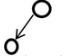This simple graph can be coloured with 2 colours. 2) n = 3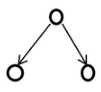Here, in this graph let us suppose vertex A is coloured with C1 and vertices B, C can be coloured with colour C2 => chromatic number is 2 In the same way, you can check with other values, Chromatic number is equals to 2

A simple graph with no odd cycles is bipartite graph and a Bipartite graph can be colored using 2 colors (See this)

QUESTION: 11

Which one of the following is TRUE for any simple connected undirected graph with more than 2 vertices?

Solution:

Since the graph is simple, there must not be any self loop and parallel edges. Since the graph is connected, the degree of any vertex cannot be 0. Therefore, degree of all vertices should be be from 1 to n-1. So the degree of at least two vertices must be same.

QUESTION: 12

Which of the following statements is true for every planar graph on n vertices?

Solution:

A planar graph is a graph which can drawn on a plan without any pair of edges crossing each other. A) FALSE: A disconnected graph can be planar as it can be drawn on a plane without crossing edges. B) FALSE: An Eulerian Graph may or may not be planar.An undirected graph is eulerian if all vertices have even degree. For example, the following graph is Eulerian, but not planar C) TRUE: D) FALSE: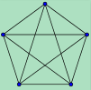QUESTION: 13

G is a graph on n vertices and 2n - 2 edges. The edges of G can be partitioned into two edge-disjoint spanning trees. Which of the following is NOT true for G?

Solution:

Counter for option D is as follows. Take two copies of K4(complete graph on 4 vertices), G1 and G2. Let V(G1)={1,2,3,4} and V(G2)={5,6,7,8}. Construct a new graph G3 by using these two graphs G1 and G2 by merging at a vertex, say merge (4,5). The resultant graph is two edge connected, and of minimum degree 2 but there exist a cut vertex, the merged vertex.

QUESTION: 14

Let G be the non-planar graph with the minimum possible number of edges. Then G has

Solution:

Explanation: According to Kuratowski’s Theorem, a graph is planar if and only if it does not contain any subdivisions of the graphs K5 or K3,3.

That means K5 and K3,3 are minimum non-planar graphs. These graphs have 5 vertices with 10 edges in K5 and 6 vertices with 9 edges in K3,3 graph.
So, graph K5 has minimum vertices and maximum edges than K3,3.

Alternative method:
A plane graph having ‘n’ vertices, cannot have more than ‘2*n-4’ number of edges. Hence using the logic we can derive that for 6 vertices, 8 edges is required to make it a plane graph. So adding one edge to the graph will make it a non planar graph.

So, 6 vertices and 9 edges is the correct answer.

QUESTION: 15

Which of the following graphs has an Eulerian circuit?

Solution:

A graph has Eulerian Circuit if following conditions are true. ….a) All vertices with non-zero degree are connected. We don’t care about vertices with zero degree because they don’t belong to Eulerian Cycle or Path (we only consider all edges). ….b) All vertices have even degree. Let us analyze all options. A) Any k-regular graph where k is an even number. is not Eulerian as a k regular graph may not be connected (property b is true, but a may not) B) A complete graph on 90 vertices is not Eulerian because all vertices have degree as 89 (property b is false) C) The complement of a cycle on 25 vertices is Eulerian. In a cycle of 25 vertices, all vertices have degree as 2. In complement graph, all vertices would have degree as 22 and graph would be connected.

QUESTION: 16

Let G=(V,E) be a directed graph where V is the set of vertices and E the set of edges. Then which one of the following graphs has the same strongly connected components as G ?

Solution:

If we reverse directions of all arcs in a graph, the new graph has same set of strongly connected components as the original graph. Se

QUESTION: 17

Consider an undirected graph G where self-loops are not allowed. The vertex set of G is {(i, j): 1 <= i <= 12, 1 <= j <= 12}. There is an edge between (a, b) and (c, d) if |a − c| <= 1 and |b − d| <= 1. The number of edges in this graph is __________.

Solution:

Given: The vertex set of G is {(i, j): 1 <= i <= 12, 1 <= j <= 12}. There is an edge between (a, b) and (c, d) if |a − c| <= 1 and |b − d| <= 1.
There can be total 12*12 possible vertices. The vertices are (1, 1), (1, 2) ....(1, 12) (2, 1), (2, 2), ....
The number of edges in this graph? Number of edges is equal to number of pairs of vertices that satisfy above conditions.For example, vertex pair {(1, 1), (1, 2)} satisfy above condition.
For (1, 1), there can be an edge to (1, 2), (2, 1), (2, 2). Note that there can be self-loop as mentioned in the question. Same is count for (12, 12), (1, 12) and (12, 1)
For (1, 2), there can be an edge to (1, 1), (2, 1), (2, 2), (2, 3), (1, 3)
Same is count for (1, 3), (1, 4)....(1, 11), (12, 2), ....(12, 11) For (2, 2), there can be an edge to (1, 1), (1, 2), (1, 3), (2, 1), (2, 3), (3, 1), (3, 2), (3, 3)
Same is count for remaining vertices. For all pairs (i, j) there can total 8 vertices connected to them if i and j are not in {1, 12} There are total 100 vertices without a 1 or 12. So total 800 edges. For vertices with 1, total edges = (Edges where 1 is first part) + (Edges where 1 is second part and not first part) =
(3 + 5*10 + 3) + (5*10) edges Same is count for vertices with 12
Total number of edges:
= 800 + [(3 + 5*10 + 3) + 5*10] + [(3 + 5*10 + 3) + 5*10]
= 800 + 106 + 106
= 1012
Since graph is undirected, two edges from v1 to v2 and v2 to v1 should be counted as one.
So total number of undirected edges = 1012/2 = 506.

QUESTION: 18

An ordered n-tuple (d1, d2, … , dn) with d1 >= d2 >= ⋯ >= dn is called graphic if there exists a simple undirected graph with n vertices having degrees d1, d2, … , dn respectively. Which of the following 6-tuples is NOT graphic?

Solution:

The required graph is not possible with the given degree set of (3, 3, 3, 1, 0, 0). Using this 6-tuple the graph formed will be a Disjoint undirected graph, where the two vertices of the graph should not be connected to any other vertex ( i.e. degree will be 0 for both the vertices ) of the graph. And for the remaining 4 vertices the graph need to satisfy the degrees of (3, 3, 3, 1).

Let's see this with the help of a logical structure of the graph :

Let's say vertices labelled as <ABCDEF> should have their degree as <3, 3, 3, 1, 0, 0> respectively.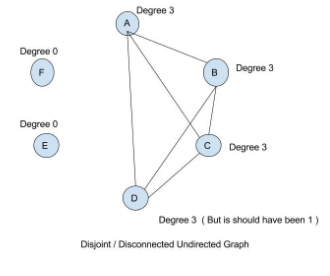Now E and F should not be connected to any vertex in the graph. And A, B, C and D should have their degree as <3, 3, 3, 1> respectively. Now to fulfill the requirement of A, B and C, the node D will never be able to get its degree as 1. It's degree will also become as 3. This is shown in the above diagram.

Hence tuple <3, 3, 3, 1, 0, 0> is not graphic.

*Answer can only contain numeric values
QUESTION: 19

The maximum number of edges in a bipartite graph on 12 vertices is

Solution:

Number of edges would be maximum when there are 6 edges on each side and every vertex is connected to all 6 vertices of the other side.

QUESTION: 20

A cycle on n vertices is isomorphic to its complement. The value of n is _____

Solution:

Below is a cyclic graph with 5 vertices and its complement graph.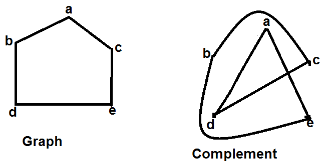The complement graph is also isomorphic (same number of vertices connected in same way) to given graph.